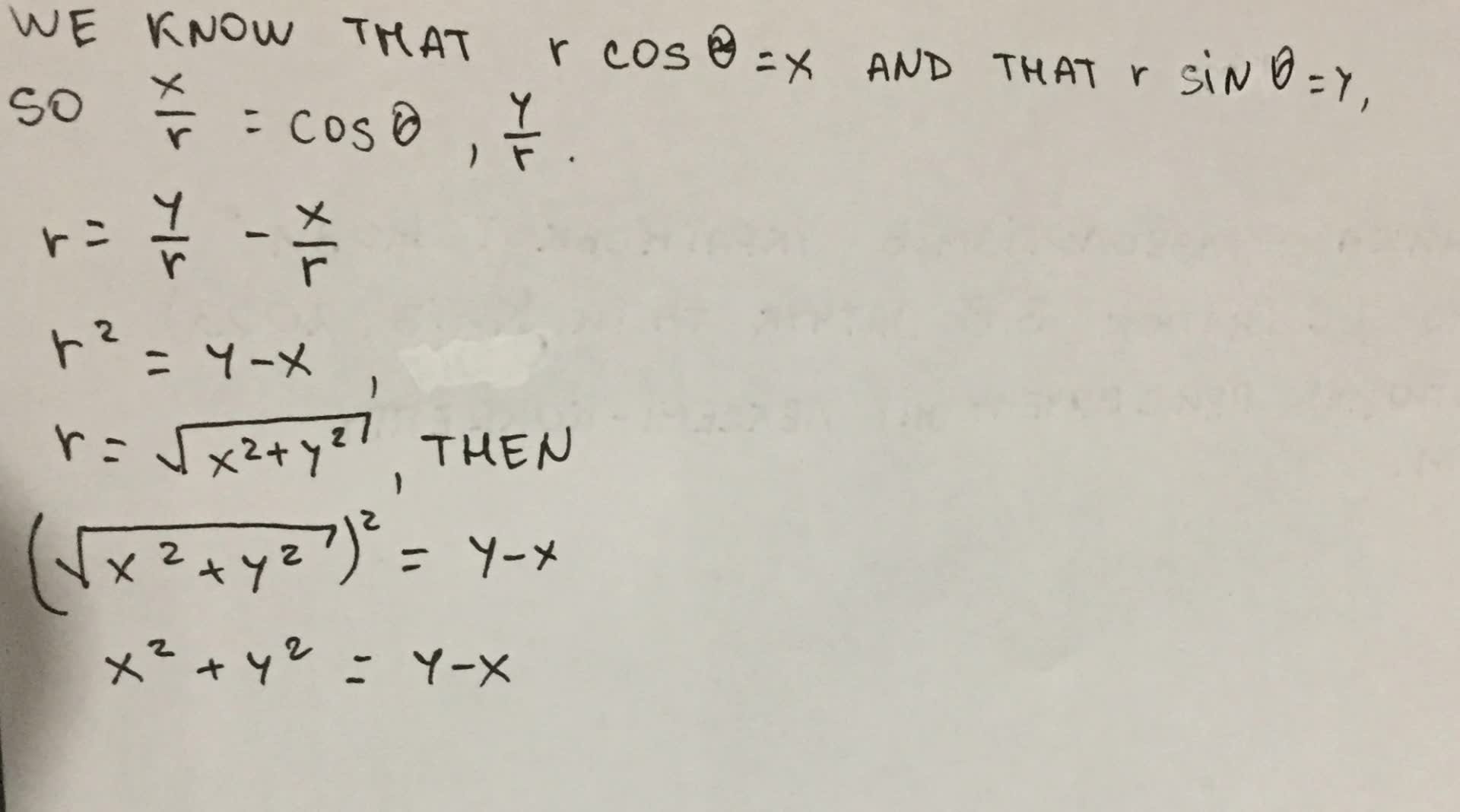The letters r and theta represent polar coordinates. Write each equation using rectangular coordinates. r=sin theta-cos theta r=sin theta-cos theta.Lipossig 2021-09-05 Answered

The letters $$\displaystyle{r}{\quad\text{and}\quad}θ$$ represent polar coordinates. Write each equation using rectangular coordinates $$(x, y)$$.

$$\displaystyle{r}= \sin{\theta}- \cos{\theta}{r}= \sin{\theta}- \cos{\theta}$$

• Questions are typically answered in as fast as 30 minutes

Solve your problem for the price of one coffee

• Math expert for every subject
• Pay only if we can solve itberggansS

We know that $$\displaystyle{r} \cos{\theta}={x}$$ and that $$\displaystyle{r} \sin{\theta}={y}$$, so $$\displaystyle\frac{x}{{r}}= \cos{\theta}{\quad\text{and}\quad}\frac{y}{{r}}.$$
Thus, $$\displaystyle{r}=\frac{y}{{r}}–\frac{x}{{r}}.$$
Multiplying both sides by r gives:
$$\displaystyle{R}^{2}={y}–{x}.$$
We know that $$\displaystyle{r}=\sqrt{{{x}^{2}+{y}^{2}}}$$, then
$$\displaystyle{x}^{2}+{y}^{2}={y}-{x}$$